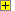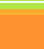Physics GCSE
Unit 2 (electricity)Calculations based on formulae in AQA's GCSE Physics Unit 2 (electricity section).
As well as random selection of the formula, the equation is randomly re-arranged.Show Calculator